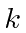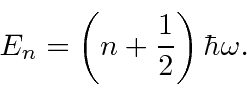The Harmonic Oscillator in One Dimension

Next we solve for the energy eigenstates of the harmonic oscillator potential, where we have eliminated the spring constantby using the classical oscillator frequency. The energy eigenvalues areThe energy eigenstates turn out to be a polynomial (in) of degreetimes. So the ground state, properly normalized, is justWe will later return the harmonic oscillator to solve the problem by operator methods.

Jim Branson 2013-04-22